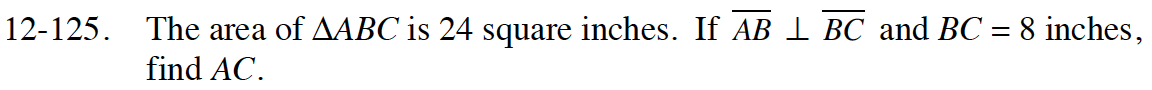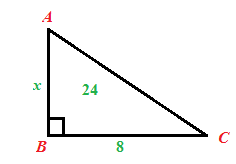Home > CCA2 > Chapter 12 > Lesson 12.2.2 > Problem12-125

12-125.

The area of ∆ABC is 24 square inches. If ABBC and BC = 8 inches, find AC. Homework Help ✎Begin by drawing and labeling a diagram.Use an area equation.

$\frac{8\textit{x}}{2}=\ 24$

x = 6 inches, what is the length of AC?